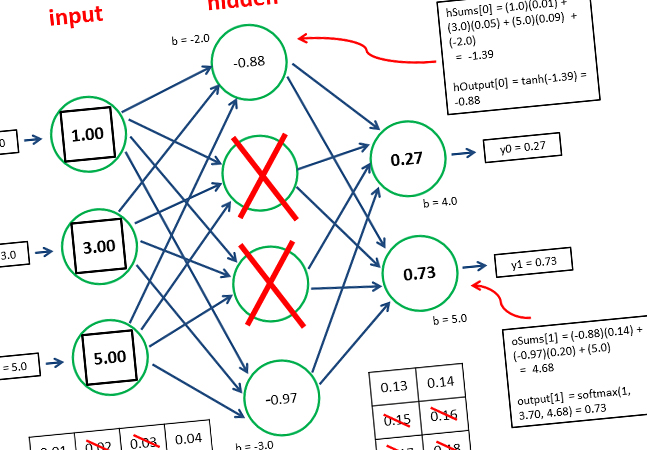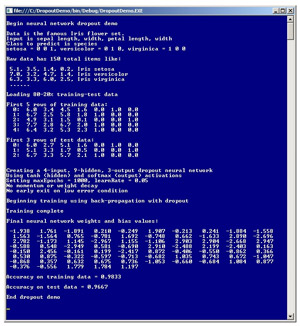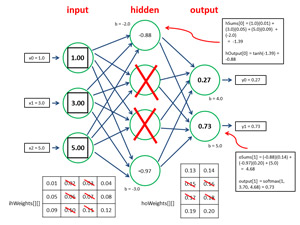#Neural Network Lab

### Neural Network Dropout Training

Dropout training is a relatively new algorithm which appears to be highly effective for improving the quality of neural network predictions. Dropout training is not yet widely implemented in neural network API libraries. The information presented in this article will enable you to understand how to use dropout training if it's available in an existing system, or add dropout training to systems where it's not yet available.A major challenge when working with a neural network is training the network in such a way that the resulting model doesn't over-fit the training data -- that is, generate weights and bias values that predict the dependent y-values of the training data with very high accuracy, but predict the y-values for new data with poor accuracy. One interesting approach for dealing with neural network over-fitting is a technique called dropout training. The idea is simple: During the training process, hidden nodes and their connections are randomly dropped from the neural network. This prevents the hidden nodes from co-adapting with each other, forcing the model to rely on only a subset of the hidden nodes. This makes the resulting neural network more robust. Another way of looking at dropout training is that dropout generates many different virtual subsets of the original neural network and then these subsets are averaged to give a final network that generalizes well.

Take a look at the demo run in Figure 1. The demo program creates and trains a neural network classifier that predicts the species of an iris flower (setosa, versicolor or virginica) based on four numeric x-values for sepal length and width and petal length and width. The training set consists of 120 data items. The 4-9-3 neural network uses the back-propagation training algorithm combined with dropout. After training, the resulting neural network model with (4 * 9) + (9 * 3) + (9 + 3) = 75 weights and bias values correctly predicts the species of 29 of the 30 data items (0.9667 accuracy) in the test set. The dropout process occurs behind the scenes.[Click on image for larger view.] Figure 1. Neural Network Training Using Dropout

This article assumes you have a solid understanding of neural network concepts, including the feed-forward mechanism, and the back-propagation algorithm, and that you have at least intermediate level programming skills, but does not assume you know anything about dropout training. The demo is coded using C# but you should be able to refactor the code to other languages such as JavaScript or Visual Basic .NET without too much difficulty. The demo code is too long to present in its entirety, so this article focuses on the methods that use dropout. Most normal error checking has been omitted from the demo to keep the main ideas as clear as possible.

Overall Program Structure
The demo program is a console application. The overall structure of the program, with some minor edits and WriteLine statements removed, is presented in Listing 1. Compared to a neural network class that doesn't use dropout, the neural network code in Listing 1 has additional methods MakeDropNodes and IsDropNode, and methods ComputeOutputs and UpdateWeights have an additional input parameter array named dropNodes.

Listing 1: Dropout Training Demo Program Structure
```using System;
using System.Collections.Generic;
namespace DropoutDemo
{
class DropoutProgram
{
static void Main(string[] args)
{
Console.WriteLine("\nBegin neural network dropout demo");
double[][] trainData = new double[];
trainData = new double[] { 6.0,3.4,4.5,1.6, 0,1,0 };
// Etc. ...
trainData = new double[] { 5.7,2.8,4.5,1.3, 0,1,0 };

double[][] testData = new double[];
testData = new double[] { 6.0,2.7,5.1,1.6, 0,1,0 };
// Etc. ...
testData = new double[] { 5.8,2.6,4.0,1.2, 0,1,0 };

Console.WriteLine("\nFirst 5 rows of training data:");
ShowMatrix(trainData, 5, 1, true);
Console.WriteLine("First 3 rows of test data:");
ShowMatrix(testData, 3, 1, true);

const int numInput = 4;
const int numHidden = 7;
const int numOutput = 3;
NeuralNetwork nn = new NeuralNetwork(numInput,
numHidden, numOutput);

int maxEpochs = 500;
double learnRate = 0.05;
nn.Train(trainData, maxEpochs, learnRate);
Console.WriteLine("Training complete\n");

double[] weights = nn.GetWeights();
Console.WriteLine("Final weights and bias values:");
ShowVector(weights, 10, 3, true);

double trainAcc = nn.Accuracy(trainData);
Console.WriteLine("\nAccuracy on training data = " +
trainAcc.ToString("F4"));

double testAcc = nn.Accuracy(testData);
Console.WriteLine("\nAccuracy on test data = " +
testAcc.ToString("F4"));

Console.WriteLine("\nEnd dropout demo\n");
}

static void ShowVector(double[] vector, int valsPerRow,
int decimals, bool newLine) { . . }
static void ShowMatrix(double[][] matrix, int numRows,
int decimals, bool newLine) { . . }
} // class Program

public class NeuralNetwork
{
private static Random rnd;

private int numInput;
private int numHidden;
private int numOutput;

private double[] inputs;
private double[][] ihWeights; // input-hidden
private double[] hBiases;
private double[] hOutputs;

private double[][] hoWeights; // hidden-output
private double[] oBiases;
private double[] outputs;

public NeuralNetwork(int numInput, int numHidden,
int numOutput) { . . }
private static double[][] MakeMatrix(int rows,
int cols) { . . }
public void SetWeights(double[] weights) { . . }
private void InitializeWeights() { . . }
public double[] GetWeights() { . . }

private int[] MakeDropNodes() { . . }
private bool IsDropNode(int node, int[] dropNodes) { . . }

private double[] ComputeOutputs(double[] xValues,
int[] dropNodes) { . . }
private static double HyperTanFunction(double x) { . . }
private static double[] Softmax(double[] oSums) { . . }

private void UpdateWeights(double[] tValues,
double learnRate, int[] dropNodes) { . . }

public void Train(double[][] trainData, int maxEprochs,
double learnRate) { . . }
private static void Shuffle(int[] sequence) { . . }
public double Accuracy(double[][] testData) { . . }
private static int MaxIndex(double[] vector) { . . }
} // class NeuralNetwork
} // ns```

The Dropout Process
Although neural network training using dropout is conceptually simple, the implementation details are a bit tricky. Take a look at the diagram in Figure 2. The diagram represents a dummy neural network that has three inputs, four hidden nodes and two outputs. When using dropout, as each training data item is presented, some of the hidden nodes are randomly selected to be dropped just for the current training item. Specifically, each node independently has a probability equal to 0.50 of being dropped. This means that no hidden nodes might be selected to be dropped, or all hidden nodes might be selected, but on average, about half of the hidden nodes will be selected as drop-nodes for each training item.[Click on image for larger view.] Figure 2. Effect of Dropout Nodes on Feed-Forward

In Figure 2, hidden nodes  and  were selected to be dropped. The dropped nodes do not participate in the feed-forward computation of the output values, or in the back-propagation computation to update the neural network weights and bias values.

The figure shows how the two dropped nodes affect the feed-forward computation. Hidden node  isn't a drop-node, so its hSums value is computed as normal. If the input-to-hidden weights are:

```0.01  0.02  0.03  0.04
0.05  0.06  0.07  0.08
0.09  0.10  0.11  0.12```

and the three hidden node bias value are -2.0, -2.3, -2.6 and -3.0, and the input x-values are 1.0, 3.0, 5.0, then hSums = (1.0)(0.01) + (3.0)(0.05) + (5.0)(0.09) + (-2.0) = -1.39. If the tanh function is used for hidden node activation, the output for hidden node  = tanh(-1.39) = -0.88. The hSums and output values for hidden nodes  and  aren't computed for the current training item because those nodes have been selected as drop-nodes for the training item. Note that hidden nodes selected to be dropped aren't physically removed from the neural network, they're virtually removed by ignoring them.

After hidden node output values are computed, ignoring the drop-nodes, the final output values of the neural network are computed in much the same way. For example, in Figure 2, if the hidden-to-output weights are:

```0.13  0.14
0.15  0.16
0.17  0.18
0.19  0.20```

and the two output node bias values are 4.0 and 5.0, and the outputs for hidden nodes  and  are -0.88 and -0.97, then oSums = (-0.88)(0.13) + (-0.97)(0.19) + 4.0 = 3.70. And oSums = (-0.88)(0.14) + (-0.97)(0.20) + 5.0 = 4.68. If the softmax function is used for output layer activation, then the two final outputs of the neural network are softmax(0, 3.70, 4.68) = 0.27 and softmax(1, 3.70, 4.68) = 0.73. In short, those hidden nodes selected to be dropped for the current training item are ignored.

The effect of drop-nodes on the back-propagation pass through the neural network is similar. Hidden node gradients aren't computed for the drop-nodes, and the drop-node input-hidden weights, hidden-output weights, and hidden biases aren't updated.

Generating Nodes to Drop
As each training item is presented for training, a new set of randomly selected hidden nodes to drop must be generated. Method MakeDropNodes returns an array of type int where the values in the array are indices of drop-nodes. For example, if a neural network has nine hidden nodes and the return from MakeDropNodes is an array of size four with values 0, 2, 6, 8 then there are four drop-nodes at indices , ,  and . Method MakeDropNodes is defined in Listing 2.

Listing 2: The MakeDropNodes Method
```private int[] MakeDropNodes()
{
List<int> resultList = new List<int>();
for (int i = 0; i < this.numHidden; ++i) {
double p = rnd.NextDouble();
}

if (resultList.Count == 0)
else if (resultList.Count == numHidden)
resultList.RemoveAt(rnd.Next(0, numHidden));
return resultList.ToArray();
}```

The method iterates once for each hidden node. A random value between 0.0 and 1.0 is generated, and if that value is less than 0.5 (a 0.50 probability), the current hidden node's index is added to the result list of drop-nodes. The method checks to see if no hidden nodes were generated, and if so, one randomly selected node is chosen. Also, if all hidden nodes were selected as drop-nodes, one randomly selected node is removed. Notice that this process generates an array of drop-node indices that are in order from low to high.

After an array of drop-nodes has been generated, during training, a hidden node can be determined to be a drop-node or not using this function:

```private bool IsDropNode(int node, int[] dropNodes)
{
if (dropNodes == null)
return false;

if (Array.BinarySearch(dropNodes, node) >= 0)
return true;
else
return false;
}```

Because the drop-nodes are in order, the efficient BinarySearch method can be used to search the array of drop-nodes. An alternative design for neural networks -- with a very large number of hidden nodes -- is to store drop-nodes in a Hashtable collection rather than in an array. Searching the Hashtable can be done in linear time as opposed to the binary search, which operates in log(n) time.

If a variable j holds the index of a hidden node, then determining if j is a drop-node can be done using this statement:

`if (IsDropNode(j, dropNodes) == true) // j is a drop-node ... `

Dropping hidden nodes with probability 0.50 is the most common approach in dropout training but you may want to experiment with other probability values.

Feed-Forward with Dropout
The demo program performs the feed-forward process to compute y-values for a given set of x-values using method ComputeOutputs. The method's definition begins:

```private double[] ComputeOutputs(double[] xValues, int[] dropNodes)
{
if (xValues.Length != numInput)
throw new Exception("Bad xValues array length");

double[] hSums = new double[numHidden];
double[] oSums = new double[numOutput];

for (int i = 0; i < xValues.Length; ++i)
this.inputs[i] = xValues[i];
...```

After a rudimentary input parameter check, the scratch sums (before activation is applied) arrays are allocated and the input x-values are copied into the neural network's inputs array without any modification. Next, the hidden node outputs are computed:

```for (int j = 0; j < numHidden; ++j)  // Each hidden node
{
if (IsDropNode(j, dropNodes) == true) continue; // skip
for (int i = 0; i < numInput; ++i)
hSums[j] += this.inputs[i] * this.ihWeights[i][j];

hSums[j] += this.hBiases[j]; // Add bias

this.hOutputs[j] = HyperTanFunction(hSums[j]); // Apply activation
}
...```

As just described, the hidden sums are computed as usual, except each hidden node is first checked to see if it's a drop-node for the current training item. Then the bias values are added, and the tanh activation function is applied next. The output node values are computed using the same logic:

```for (int k = 0; k < numOutput; ++k)   // Each output node
{
for (int j = 0; j < numHidden; ++j)
{
if (IsDropNode(j, dropNodes) == true) continue; // Skip
oSums[k] += hOutputs[j] * hoWeights[j][k];
}

oSums[k] += oBiases[k]; // Add bias

double[] softOut = Softmax(oSums);
Array.Copy(softOut, outputs, softOut.Length);
}
...```

Method ComputeOutputs concludes by adding output biases, applying the softmax activation function, and copying the final output y-values from the internal outputs array to a return array copy of the values:

```...
for (int k = 0; k < numOutput; ++k) // Add biases
oSums[k] += oBiases[k];

double[] softOut = Softmax(oSums);
Array.Copy(softOut, outputs, softOut.Length);

double[] retResult = new double[numOutput];
Array.Copy(this.outputs, retResult, retResult.Length);
return retResult;
}```

To summarize, computing neural network outputs using dropout can be performed by checking each hidden node during the feed-forward algorithm to see if it's a drop-node, and if so, simply skipping that node.

Back-Propagation with Dropout
Updating a neural network's weights and bias values during back-propagation follows the same pattern used when computing outputs. In the demo, the back-propagation process is implemented in method UpdateWeights. The method begins by computing the output gradients:

```private void UpdateWeights(double[] tValues, double learnRate, int[] dropNodes)
{

for (int k = 0; k < numOutput; ++k) // Output gradients
{
// Implicit MSE
double derivative = (1 - outputs[k]) * outputs[k];
oGrads[k] = derivative * (tValues[k] - outputs[k]);
}
. . .```

Next, the hidden node gradients are computed, but with drop-nodes skipped:

```for (int j = 0; j < numHidden; ++j)
{
if (IsDropNode(j, dropNodes) == true) continue;
double derivative = (1 - hOutputs[j]) * (1 + hOutputs[j]);
double sum = 0.0;
for (int k = 0; k < numOutput; ++k)
{
double x = oGrads[k] * hoWeights[j][k];
sum += x;
}
}
...```

Next, the input-to-hidden weights and hidden biases are updated. For processing efficiency, the two logically separate tasks are combined into a single pair of nested loops:

```for (int j = 0; j < numHidden; ++j)
{
if (IsDropNode(j, dropNodes) == true) continue;
for (int i = 0; i < numInput; ++i)
{
double delta = learnRate * hGrads[j] * inputs[i];
ihWeights[i][j] += delta;
}
double biasDelta = learnRate * hGrads[j] * 1.0; // dummy 1.0
hBiases[j] += biasDelta;
}
...```

Method UpdateWeights concludes by updating the hidden-to-output weights and the output biases in a single pair of nested loops:

```...
for (int k = 0; k < numOutput; ++k)
{
for (int j = 0; j < numHidden; ++j)
{
if (IsDropNode(j, dropNodes) == true) continue;
double delta = learnRate * oGrads[k] * hOutputs[j];
hoWeights[j][k] += delta;
}
double biasDelta = learnRate * oGrads[k] * 1.0;
oBiases[k] += biasDelta;
}
}```

Adjusting the Final Hidden-to-Output Weight Values
When using dropout training, it's necessary to adjust the hidden-to-output weights after training has completed, because the final neural network model is effectively using twice as many hidden nodes as were used to create the model. In the demo program this halving is performed in method Train. Method Train begins by setting up arrays to hold the input x-values and the target y-values, and by preparing a sequence of index value into the training data so that the training items can be presented in a random order:

```public void Train(double[][] trainData, int maxEprochs, double learnRate)
{
int epoch = 0;
double[] xValues = new double[numInput]; // Inputs
double[] tValues = new double[numOutput]; // Targets

int[] sequence = new int[trainData.Length];
for (int i = 0; i < sequence.Length; ++i)
sequence[i] = i;
... ```

The main training loop generates drop-nodes and repeatedly performs the feed-forward and back-propagation processes. Note that method Train assumes the encoded target values are located in the last numOutput columns of the trainData matrix.

```while (epoch < maxEpochs)
{
Shuffle(sequence); // visit each training data in random order
for (int i = 0; i < trainData.Length; ++i)
{
int idx = sequence[i];
Array.Copy(trainData[idx], xValues, numInput);
Array.Copy(trainData[idx], numInput, tValues, 0, numOutput);
int[] dropNodes = MakeDropNodes();
ComputeOutputs(xValues, dropNodes);
UpdateWeights(tValues, learnRate, dropNodes);
} // each training item
++epoch;
}
...```

An alternative design is to store the dropNodes array as a NeuralNetwork class member instead of a separate method-scope array. Method Train finishes by reducing the hidden-to-output weight values by a factor of two:

```...
for (int j = 0; j < numHidden; ++j)
for (int k = 0; k < numOutput; ++k)
hoWeights[j][k] /= 2.0;
} // Train```

The demo program uses hardcoded drop probability and associated reduction factor of 0.50 and 2.0, respectively. An alternative design is to parameterize these values. For example, if you specified a drop probability of 1/3 = 0.33, the reduction factor would be 3.0.

Dealing With Over-Fitting
Neural network dropout training is a relatively new technique for dealing with over-fitting. This article is based on the 2012 research paper titled "Improving Neural Networks by Preventing Co-Adaptation of Feature Detectors." Although experience with dropout is limited, the research suggests the technique can be part of an effective strategy to limit over-fitting and generate accurate classifiers. It isn't clear how dropout training interacts with other related techniques such as weight decay and weight restriction. This article used dropout in conjunction with back-propagation training. An unexplored but promising possibility is to use dropout with particle swarm optimization training.